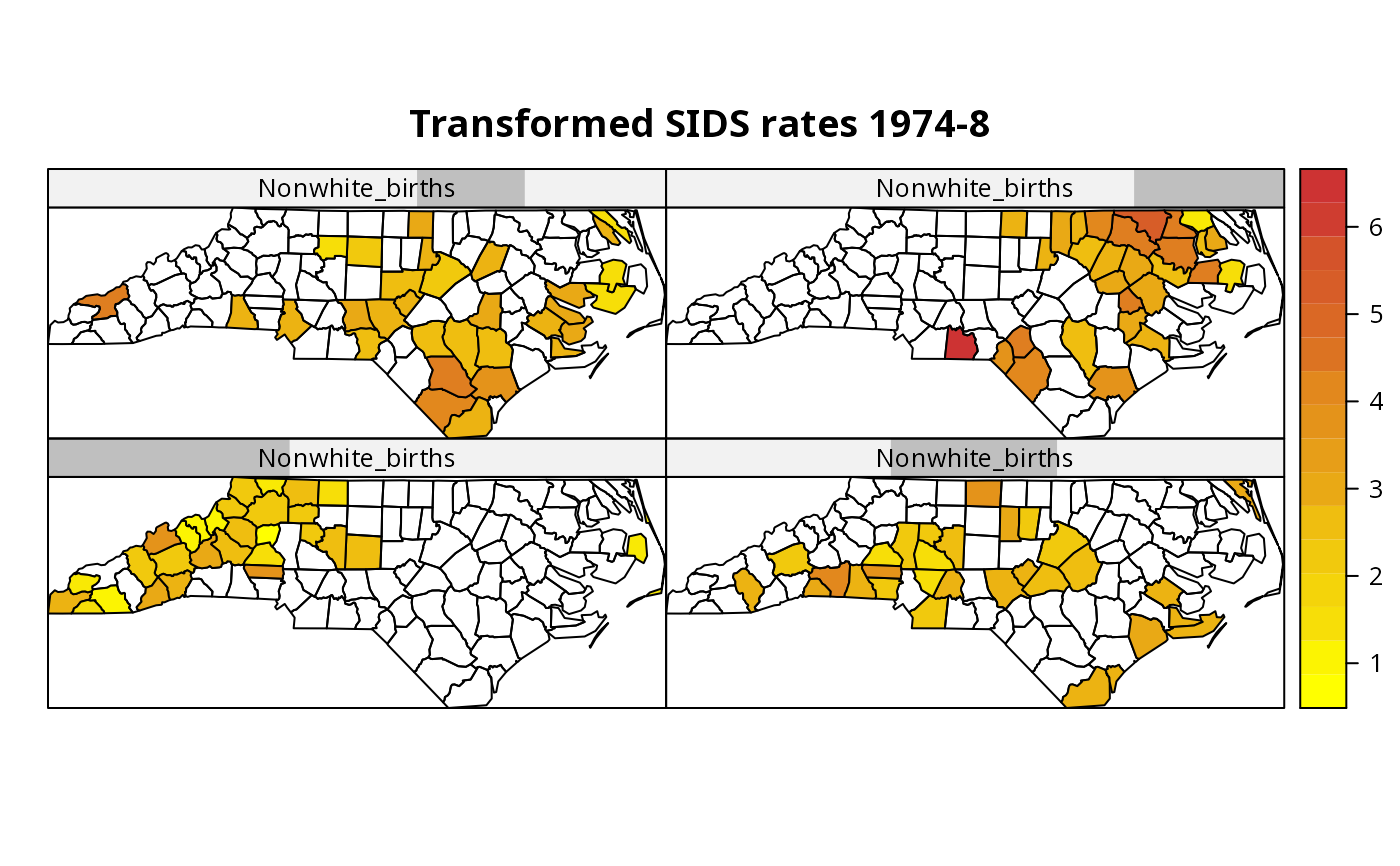Conditioned choropleth maps permit the conditioning of a map of a variable on the values of one or two other variables coded as factors or shingles. This function uses spplot after constructing multiple subsets of the variable of interest defined by the intervals given by the conditioning variables.

CCmaps(obj, zcol = NULL, cvar = NULL, cvar.names = NULL, ..., names.attr,
scales = list(draw = FALSE), xlab = NULL, ylab = NULL,
aspect = mapasp(obj, xlim, ylim), sp.layout = NULL, xlim = bbox(obj)[1, ],
ylim = bbox(obj)[2, ])

## Arguments

obj object of class SpatialPolygonsDataFrame single variable name as string a list of one or two conditioning variables, which should be of class factor or shingle names for conditioning variables, if not given, the names of the variables in the cvar list other arguments passed to spplot and levelplot names to use in panel, if different from zcol names scales argument to be passed to Lattice plots; use list(draw = TRUE) to draw axes scales label for x-axis label for y-axis aspect ratio for spatial axes; defaults to "iso" (one unit on the x-axis equals one unit on the y-axis) but may be set to more suitable values if the data are e.g. if coordinates are latitude/longitude NULL or list; see spplot numeric; x-axis limits numeric; y-axis limits

## Value

The function returns a SpatialPolygonsDataFrame object with the zcol variable and the partitions of the cvars list variables invisibly.

Carr D, Wallin J, Carr D (2000) Two new templates for epidemiology applications: linked micromap plots and conditioned choropleth maps. Statistics in Medicine 19(17-18): 2521-2538 Carr D, White D, MacEachren A (2005) Conditioned choropleth maps and hypothesis generation. Annals of the Association of American Geographers 95(1): 32-53 Friendly M (2007) A.-M. Guerry's Moral Statistics of France: challenges for multivariable spatial analysis. Statistical Science 22(3): 368-399

## Author

Roger Bivand

spplot

## Examples

nc.sids <- readShapeSpatial(system.file("shapes/sids.shp",
package="maptools"), IDvar="FIPSNO",
proj4string=CRS("+proj=longlat +ellps=clrk66"))
#> Warning: readShapeSpatial is deprecated; use rgdal::readOGR or sf::st_read#> Warning: readShapePoly is deprecated; use rgdal::readOGR or sf::st_read#> Warning: Discarded datum Unknown based on Clarke 1866 ellipsoid in Proj4 definitionnc.sids$ft.SID74 <- sqrt(1000)*(sqrt(nc.sids$SID74/nc.sids$BIR74) + sqrt((nc.sids$SID74+1)/nc.sids$BIR74)) nc.sids$ft.NWBIR74 <- sqrt(1000)*(sqrt(nc.sids$NWBIR74/nc.sids$BIR74) +
sqrt((nc.sids$NWBIR74+1)/nc.sids$BIR74))
library(lattice)
sh_nw4 <- equal.count(nc.sids\$ft.NWBIR74, number=4, overlap=1/5)
CCmaps(nc.sids, "ft.SID74", list("Nonwhite_births"=sh_nw4),
col.regions=colorRampPalette(c("yellow1", "brown3"))(20),
main="Transformed SIDS rates 1974-8")